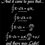# Quite weird

$\Large ∫_{-∝}^{0} log ⁡(n+e^{x})$ $n\in R$

Does any value of $n$ exists such that the above integral becomes $\Large 0$ ?Note by Akash Shukla
5 years, 1 month ago

This discussion board is a place to discuss our Daily Challenges and the math and science related to those challenges. Explanations are more than just a solution — they should explain the steps and thinking strategies that you used to obtain the solution. Comments should further the discussion of math and science.

When posting on Brilliant:

• Use the emojis to react to an explanation, whether you're congratulating a job well done , or just really confused .
• Ask specific questions about the challenge or the steps in somebody's explanation. Well-posed questions can add a lot to the discussion, but posting "I don't understand!" doesn't help anyone.
• Try to contribute something new to the discussion, whether it is an extension, generalization or other idea related to the challenge.

MarkdownAppears as
*italics* or _italics_ italics
**bold** or __bold__ bold
- bulleted- list
• bulleted
• list
1. numbered2. list
1. numbered
2. list
Note: you must add a full line of space before and after lists for them to show up correctly
paragraph 1paragraph 2

paragraph 1

paragraph 2

[example link](https://brilliant.org)example link
> This is a quote
This is a quote
    # I indented these lines
# 4 spaces, and now they show
# up as a code block.

print "hello world"
# I indented these lines
# 4 spaces, and now they show
# up as a code block.

print "hello world"
MathAppears as
Remember to wrap math in $$ ... $$ or $ ... $ to ensure proper formatting.
2 \times 3 $2 \times 3$
2^{34} $2^{34}$
a_{i-1} $a_{i-1}$
\frac{2}{3} $\frac{2}{3}$
\sqrt{2} $\sqrt{2}$
\sum_{i=1}^3 $\sum_{i=1}^3$
\sin \theta $\sin \theta$
\boxed{123} $\boxed{123}$

Sort by:

The integral doesn't exist if $n \neq 1$. If the integral exists when $n=1$, then the integral is positive as the integrand is always positive.

Hence, no value of $n$ exists such that the integral vanishes.

Edit:

$\int_{-\infty}^0 \ln (1+e^x) dx = \frac{\pi^2}{12}$

- 5 years, 1 month ago

I didn't get it. Why the integral doesn't exist for $0, as you said the integral exists only for $n=1$, Thank you.

- 5 years, 1 month ago

If $n \neq 1$, then $\lim_{x \to -\infty} \log (n+e^x) \neq 0$ and hence the integral diverges.

- 5 years, 1 month ago

I have used the series of $log$ to solve it.

$log(n+x) = log[1-(1-n-e^{x})]$,

Let, $1-n-e^{x} = A$,

So, $∫_{-∝}^{0} log ⁡(1-A) = \dfrac{A^2}{1*2}+\dfrac{A^3}{2*3}+\dfrac{A^4}{3*4}\cdots$,

Here it can be seen that it will converge for some values of $n$ which is nearer to $1$.

- 5 years, 1 month ago

You should have $-1\leq A <1$

Plus, you didn't integrate in the right way. If you did, you'll end up with infinty right from the first term.

- 5 years, 1 month ago

Yes. As $A=1-N-e^x$, so $0 and $0,because we have $-∝, will satisfy the condition.

- 5 years, 1 month ago

You didn't integrate properly. If you did, you'd have got infinity a lot of times.

- 5 years, 1 month ago

I got. But not for values of $n<1, n>0$ . Is there any mistake in the method I have shown?

- 5 years, 1 month ago

A basic test of convergence is that if $\lim_{x \to -\infty} f(x) \neq 0$, then, $\int_{-\infty}^0 f(x) dx$ diverges.

I think you've not the integration correctly.

- 5 years, 1 month ago